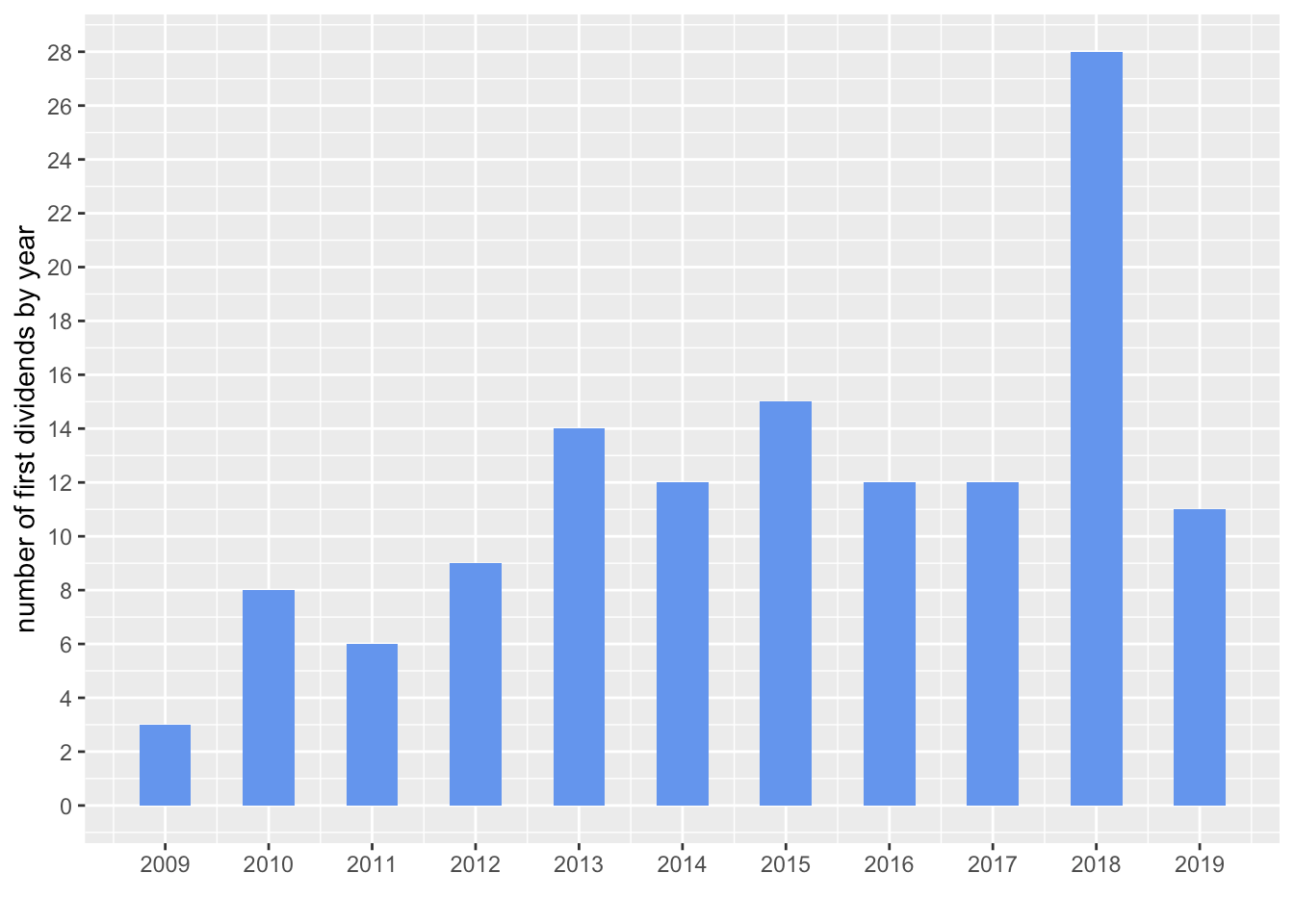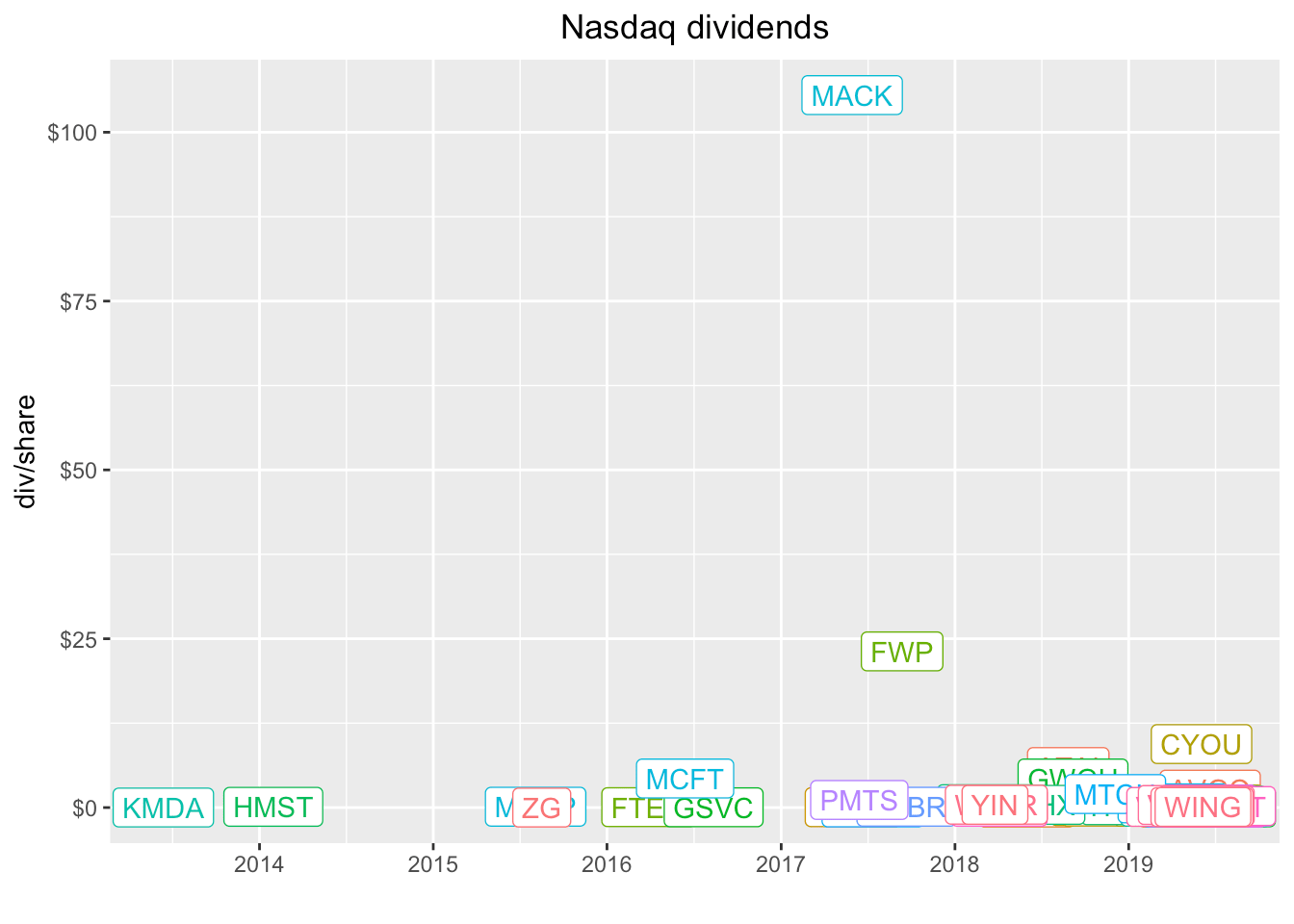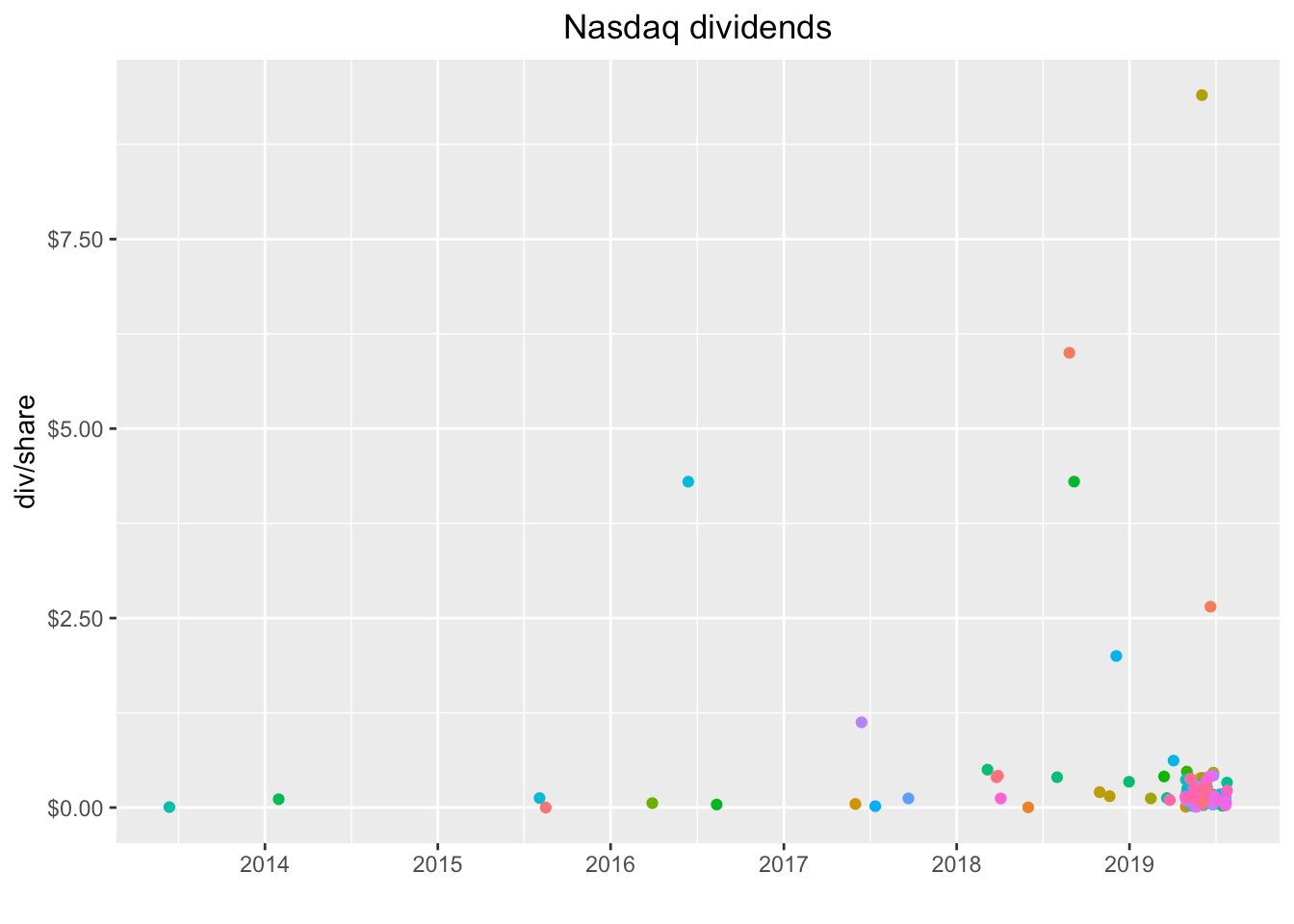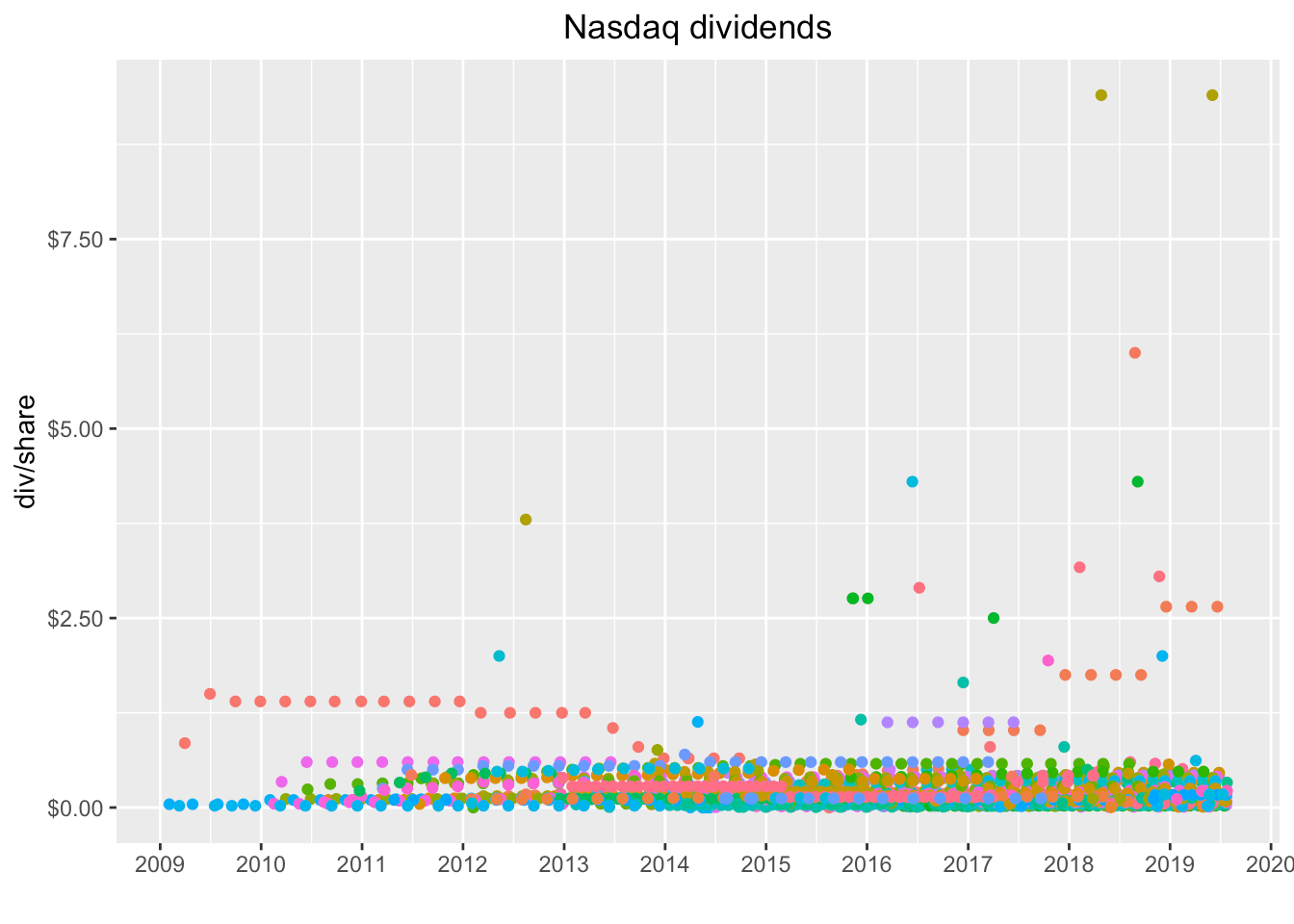Want to share your content on R-bloggers? click here if you have a blog, or here if you don't.

In a previous post, we explored the dividend history of stocks included in the SP500. Today, we’ll extend that analysis to cover the Nasdaq because, well, because in the previous post I said I would do that. We’ll also explore a different source for dividend data, do some string cleaning and check out ways to customize a tooltip in `plotly`. Bonus feature: we’ll get into some animation too. We have a lot to cover, so let’s get to it.

We need to load up our packages for the day.

```library(tidyverse)
library(tidyquant)
library(janitor)
library(plotly)```

First, we need all the companies listed on the Nasdaq. Not so long ago, it wasn’t easy to import that information into R. Now we can use `tq_exchange("NASDAQ")` from the `tidyquant` package.

```nasdaq <-
tq_exchange("NASDAQ")

nasdaq %>%

```# A tibble: 6 x 7
symbol company     last.sale.price market.cap ipo.year sector  industry
<chr>  <chr>                 <dbl> <chr>         <dbl> <chr>   <chr>
1 YI     111, Inc.              3.5  \$285.81M       2018 Health… Medical/N…
2 PIH    1347 Prope…            5.02 \$30.18M        2014 Finance Property-…
3 PIHPP  1347 Prope…           25.6  \$17.93M          NA Finance Property-…
4 TURN   180 Degree…            1.95 \$60.56M          NA Finance Finance/I…
5 FLWS   1-800 FLOW…           19.1  \$1.23B         1999 Consum… Other Spe…
6 BCOW   1895 Banco…            9.46 \$46.13M        2019 Finance Banks```

Notice how the `market.cap` column is of type character? Let’s coerce it to a `dbl` with `as.numeric` and while we’re at it, let’s remove the periods in all the column names with `clean_names` from the `janitor` package.

```nasdaq %>%
clean_names() %>%
mutate(market_cap = as.numeric(market_cap)) %>%
select(symbol, market_cap) %>%

```# A tibble: 6 x 2
symbol market_cap
<chr>       <dbl>
1 YI             NA
2 PIH            NA
3 PIHPP          NA
4 TURN           NA
5 FLWS           NA
6 BCOW           NA```

Not exactly what we had in mind. The presence of those `M`, `B` and `\$` characters are causing `as.numeric()` to coerce the column to NAs. If we want to do any sorting by market cap, we’ll need to clean that up and it’s a great chance to explore some `stringr`. Let’s start with `str_remove_all` and remove those non-numeric characters. The call is `market_cap %>% str_remove_all("\\\$|M|B")`, and then an `arrange(desc(market_cap))` so that the largest cap company is first.

```nasdaq %>%
clean_names() %>%
mutate(market_cap = market_cap %>% str_remove_all("\\\$|M|B") %>% as.numeric()) %>%
arrange(desc(market_cap)) %>%

```# A tibble: 6 x 7
symbol company    last_sale_price market_cap ipo_year sector  industry
<chr>  <chr>                <dbl>      <dbl>    <dbl> <chr>   <chr>
1 CIVBP  Civista B…            69      698280        NA Finance Major Banks
2 ASRVP  AmeriServ…            28.9    606900        NA Finance Major Banks
3 ESGRP  Enstar Gr…            26.7    427040        NA Finance Property-C…
4 AGNCN  AGNC Inve…            25.9    336700        NA Consum… Real Estat…
5 SBFGP  SB Financ…            16.2    243669.       NA Finance Major Banks
6 ESGRO  Enstar Gr…            26.5    116644        NA Finance Property-C…```

Wait, that looks weird, where’s AMZN and MSFT shouldn’t they be at the top of the market cap? Look closely at `market_cap` and notice it’s been coerced to a numeric value as we intended but we didn’t account for the fact that those `M` and `B` letters were abbreviating values and standing place for a whole bunch of zeroes. The first symbol above, `CIVBP`, didn’t have an `M` or `B` because it’s market cap is low, so it didn’t have any zeroes lopped off of it. We need a way to remove the `M` and the `B` account for those zeroes that got removed. Here’s how I chose to tackle this:

1. Find all the cells that do not have an `M` or a `B`, remove the `\$` sign, convert to numeric and divide by 1000.
We do that with `if_else(str_detect(market_cap, "M|B", negate = TRUE), str_remove_all(market_cap, "\\\$") %>% as.numeric() %>%`/`(1000)`.

2. Find all the cells that have a `B`, remove the `B` and the `\$` sign, convert to numeric and multiply by 1000.
We do that with `if_else(str_detect(market_cap, "B"), str_remove_all(market_cap, "\\\$|B") %>% as.numeric() %>%`*`(1000)`.

3. Find all the cells that have an `M`, remove the `M` and the `\$` sign, convert to numeric and don’t multiply or divide.
We do that with `str_remove_all(market_cap, "\\\$|M") %>% as.numeric()))`.

Here’s the full call:

```nasdaq %>%
clean_names() %>%
mutate(market_cap =
if_else(str_detect(market_cap, "M|B", negate = TRUE),
str_remove_all(market_cap, "\\\$") %>% as.numeric() %>% `/`(1000),
if_else(str_detect(market_cap, "B"),
str_remove_all(market_cap, "\\\$|B") %>% as.numeric() %>% `*`(1000),
str_remove_all(market_cap, "\\\$|M") %>% as.numeric()))) %>%
arrange(desc(market_cap))```

```# A tibble: 3,507 x 7
symbol company   last_sale_price market_cap ipo_year sector  industry
<chr>  <chr>               <dbl>      <dbl>    <dbl> <chr>   <chr>
1 MSFT   Microsof…           135.    1028410.     1986 Techno… Computer S…
2 AAPL   Apple In…           197      890280      1980 Techno… Computer M…
3 AMZN   Amazon.c…          1788.     884360      1997 Consum… Catalog/Sp…
4 GOOGL  Alphabet…          1171.     812020        NA Techno… Computer S…
5 GOOG   Alphabet…          1170.     811240      2004 Techno… Computer S…
6 FB     Facebook…           185.     526400      2012 Techno… Computer S…
7 CSCO   Cisco Sy…            52.6    225170      1990 Techno… Computer C…
8 INTC   Intel Co…            47.0    208030        NA Techno… Semiconduc…
9 CMCSA  Comcast …            42.1    191210        NA Consum… Television…
10 PEP    Pepsico,…           126.     176800        NA Consum… Beverages …
# … with 3,497 more rows```

That finally looks how we were expecting, the top five by market cap are `MSFT`, `AMZN`, `GOOG`, `FB` and `CSCO`. Let’s save that as an object called `nasdaq_wrangled`.

```nasdaq_wrangled <-
nasdaq %>%
clean_names() %>%
mutate(market_cap =
if_else(str_detect(market_cap, "M|B", negate = TRUE),
str_remove_all(market_cap, "\\\$") %>% as.numeric() %>% `/`(1000),
if_else(str_detect(market_cap, "B"),
str_remove_all(market_cap, "\\\$|B") %>% as.numeric() %>% `*`(1000),
str_remove_all(market_cap, "\\\$|M") %>% as.numeric()))) %>%
arrange(desc(market_cap))```

Now, let’s dig in to the dividends paid by these Nasdaq-listed companies that have IPO’d in the last ten years. It’s a bit anticlimactic because most haven’t paid any dividends but here we go. First, let’s pull just the tickers for companies that IPO’d after 2007, by setting `filter(ipo_year > 2007)`.

```nasdaq_tickers <-
nasdaq_wrangled %>%
filter(ipo_year > 2007) %>%
pull(symbol)

nasdaq_tickers %>%

` "FB"   "AVGO" "TSLA" "JD"   "TEAM" "NXPI"`

We will import the dividend data using `tq_get(source = 'dividends')`, which is a wrapper for `quantmod::getDividends()` and sources dividend data from Yahoo! Finance.

We are passing 1120 symbols to this function but only those that pay a dividend will come back to us. It takes a while to run this because we still have to check on all 1120.

```nasdaq_dividends <-
nasdaq_tickers %>%
tq_get(get = 'dividends') %>%
select(-value)```

After a huge data import task like that, I like to use `slice(1)` to grab the first observation from each group, which in this case will be each `symbol`. We can count the number symbols for which we have a dividend and it’s 130.

```nasdaq_dividends %>%
group_by(symbol) %>%
slice(1) %>%
glimpse()```

```Observations: 130
Variables: 3
Groups: symbol 
\$ symbol    <chr> "AGNC", "AMAL", "ATAI", "AVGO", "AY", "BKEP", "BLMN", …
\$ date      <date> 2009-03-31, 2018-11-15, 2011-06-28, 2010-12-13, 2014-…
\$ dividends <dbl> 0.850, 0.060, 0.430, 0.070, 0.037, 0.110, 0.060, 0.003…```

We could also get a sense for how these first dividend payments cluster into years by using `count(year)`. Note we need to `ungroup()` first.

```nasdaq_dividends %>%
group_by(symbol) %>%
slice(1) %>%
mutate(year = year(date)) %>%
ungroup() %>%
count(year)```

```# A tibble: 11 x 2
year     n
<dbl> <int>
1  2009     3
2  2010     8
3  2011     6
4  2012     9
5  2013    14
6  2014    12
7  2015    15
8  2016    12
9  2017    12
10  2018    28
11  2019    11```

And a chart will help to communicate these yearly frequencies.

```nasdaq_dividends %>%
group_by(symbol) %>%
slice(1) %>%
mutate(year = year(date)) %>%
ungroup() %>%
count(year) %>%
ggplot(aes(year, n)) +
geom_col(fill = "cornflowerblue", width = .5) +
scale_x_continuous(breaks = 2008:2019) +
scale_y_continuous(breaks = scales::pretty_breaks(n = 15)) +
labs(y = "number of first dividends by year", x = "")```Hmmm, interesting. I expected the numbers to steadily increase year by year as companies became more mature and cash flow positive, but that is not the pattern we see in that plot

Now, let’s create a quick chart of the last dividend paid by each of these 130 companies, using `slice(n())`. This time we’ll plot a dot with `geom_point()`.

```nasdaq_dividends %>%
group_by(symbol) %>%
slice(n()) %>%
mutate(year = year(date)) %>%
ggplot(aes(x = year, y = dividends, color = symbol)) +
geom_point() +
scale_y_continuous(labels = scales::dollar)  +
scale_x_continuous(breaks = 2008:2019) +
labs(x = "", y = "div/share", title = "Nasdaq dividends") +
theme(legend.position = "none",
plot.title = element_text(hjust = 0.5))```Not quite as useful as a lot of the dots are right on top of each other. We do see a couple of massive outliers. Let’s add the label for each symbol with `geom_label(aes(label = symbol))`.

```nasdaq_dividends %>%
group_by(symbol) %>%
slice(n()) %>%
ggplot(aes(x = date, y = dividends, color = symbol)) +
geom_point() +
geom_label(aes(label = symbol)) +
scale_y_continuous(labels = scales::dollar)  +
scale_x_date(breaks = scales::pretty_breaks(n = 10)) +
labs(x = "", y = "div/share", title = "Nasdaq dividends") +
theme(legend.position = "none",
plot.title = element_text(hjust = 0.5))```MACK and FWP paid some huge dividends. Let’s investigate.

```nasdaq_dividends %>%
filter(symbol == "MACK" | symbol == "FWP")```

```# A tibble: 2 x 3
symbol date       dividends
<chr>  <date>         <dbl>
1 MACK   2017-05-30     106.
2 FWP    2017-09-12      23.1```

These were most likely special dividends of some sort and that might be worth investigating but it’s not our project today so let’s filter those two out of the data and then recreate the plot. Let’s remove the `geom_label` so we see just the dots again, this time by date instead of year.

```nasdaq_dividends %>%
filter(symbol != "MACK" & symbol != "FWP") %>%
group_by(symbol) %>%
slice(n()) %>%
ggplot(aes(x = date, y = dividends, color = symbol)) +
geom_point() +
# geom_label(aes(label = symbol)) +
scale_y_continuous(labels = scales::dollar)  +
scale_x_date(breaks = scales::pretty_breaks(n = 10)) +
labs(x = "", y = "div/share", title = "Nasdaq dividends") +
theme(legend.position = "none",
plot.title = element_text(hjust = 0.5))```That’s a snapshot of the last dividend paid by each company and we can see the clustering a bit better. Quite a few companies paid their last dividend before 2019, which might indicate it’s not a regular dividend. Let’s check out the entire history of each company by removing `slice(n())`.

```nasdaq_dividends %>%
filter(symbol != "MACK" & symbol != "FWP") %>%
group_by(symbol) %>%
ggplot(aes(x = date, y = dividends, color = symbol)) +
geom_point() +
# geom_label(aes(label = symbol)) +
scale_y_continuous(labels = scales::dollar)  +
scale_x_date(breaks = scales::pretty_breaks(n = 10)) +
labs(x = "", y = "div/share", title = "Nasdaq dividends") +
theme(legend.position = "none",
plot.title = element_text(hjust = 0.5))```If you’re like me, you’re just itching to hover on, say, that reddish dot between \$5 and \$7 and see which company it is, maybe even click the dot and isolate it. Good news, we can wrap that chart in a call to `ggplotly()` and get part of that functionality out of the box. We will need to add the legend back to the plot by removing `legend.position = "none"`.

```library(plotly)

ggplotly(
nasdaq_dividends %>%
filter(symbol != "MACK" & symbol != "FWP") %>%
group_by(symbol) %>%
ggplot(aes(x = date, y = dividends, color = symbol)) +
geom_point() +
scale_y_continuous(labels = scales::dollar)  +
scale_x_date(breaks = scales::pretty_breaks(n = 10)) +
labs(x = "", y = "div/share", title = "Nasdaq dividends") +
theme(plot.title = element_text(hjust = 0.5))
)```